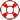Standard:

Description:

Standard:

Description:

Standard:

Description:

Standard:

Description:

Standard:

Description:

Standard:

# Math.3.MD.2 or 3.MD.A.2

Description:

## Measure and estimate liquid volumes and masses of objects using standard units of grams (g), kilograms (kg), and liters (l). Add, subtract, multiply, or divide to solve one-step word problems involving masses or volumes that are given in the same units, e.g., by using drawings (such as a beaker with a measurement scale) to represent the problem.3rd Grade Math - One-Step Problems Lesson